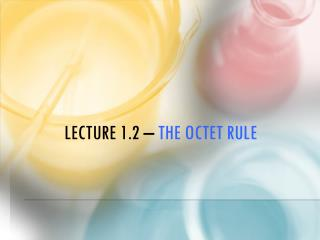DownloadDownload PresentationLecture 1.2 – the Octet Rule

# Lecture 1.2 – the Octet Rule

Download Presentation## Lecture 1.2 – the Octet Rule

- - - - - - - - - - - - - - - - - - - - - - - - - - - E N D - - - - - - - - - - - - - - - - - - - - - - - - - - -
##### Presentation Transcript

1. Lecture 1.2 – the Octet Rule

2. CA Standards • Students can determine the number of valence electrons for an element, draw a Lewis dot notation for an element and explain how the Octet rule leads to the formation of ions.

3. I. VALENCE SHORTCUT! • All elements in the same group (1A, 2A, etc.) have the same number of valence electrons. • Lewis Dot Notation shows only Valence e- • Nitrogen (#7) = • Magnesium (#12) = • Sulfur (#16) = • Sodium

4. What is the Octet Rule?

5. II. The Octet Rule • Atoms like to have a full outer valence shell  Stable atom. • gain or lose electrons to have an outer orbit with 8 electrons (or 2 if it is the first energy level). • Gain/lose based on what is easiest.

6. III. Octet SHORTCUT • All metals will losetheir valence electrons • All non-metals will gain the missing ones.

7. What are the types of ions that can form?

8. IV. Ions • When atoms gain/lose electrons, they gain a charge. • Ion– A charged atom due to unequal number of protons and electrons • Cation– A positively charged ion • Anion– A negatively charged ion

9. E- = detention hrs.  • If you gain electrons (detention hrs.)  you become negative (sad)  • If you lose electrons (detention hrs.)  you become positive (happy) 

10. CATION  POSITIVE Cats make people feel positive ANION  NEGATIVE

11. Class Example • Draw dot notation for Be. Determine the ion that forms for fluorine after the Octet Rule has been satisfied.

12. Table Talk • Draw the dot notation for Chlorine. Determine the ion that forms for nitrogen after the Octet Rule has been satisfied.

13. Stop and Jot • Draw the dot notation for Sulfur. Determine the ion that forms for oxygen after the Octet Rule has been satisfied.

14. Ion Shortcut! • All elements in the same group, form ions with the same charge.

15. MOVIE